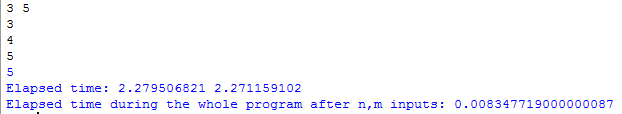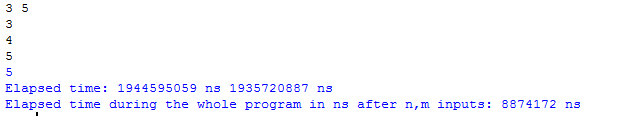# time.perf_counter() function in Python

As time module provides various time-related functions. So it is necessary to import the time module otherwise it will through error because the definition of the perf_counter() is present in time module.

perf_counter() function always returns the float value of time in seconds. Return the value (in fractional seconds) of a performance counter, i.e. a clock with the highest available resolution to measure a short duration. It does include time elapsed during sleep and is system-wide. The reference point of the returned value is undefined, so that only the difference between the results of consecutive calls is valid. In between this we can use time.sleep() and likewise fuctions.

Code #1: Understand the usage of the perf_counter .

 `# Python program to show time by perf_counter()  ` `from` `time ``import` `perf_counter ` ` `  `# integer input from user, 2 input in single line ` `n, m ``=` `map``(``int``, ``input``().split())  ` ` `  `# Start the stopwatch / counter ` `t1_start ``=` `perf_counter()  ` ` `  `for` `i ``in` `range``(n): ` `    ``t ``=` `int``(``input``()) ``# user gave input n times ` `    ``if` `t ``%` `m ``=``=` `0``: ` `        ``print``(t)  ` ` `  `# Stop the stopwatch / counter ` `t1_stop ``=` `perf_counter() ` ` `  `print``(``"Elapsed time:"``, t1_stop, t1_start)  ` ` `  ` `  `print``(``"Elapsed time during the whole program in seconds:"``, ` `                                        ``t1_stop``-``t1_start) `

Output:pref_counter_ns():
It always gives the integer value of time in nanoseconds. Similar to perf_counter(), but return time as nanoseconds.

Code #2: Usage of the perf_counter_ns and how to implement it.

 `# Python program to show time by ` `# perf_counter_ns() ` `from` `time ``import` `perf_counter_ns ` ` `  `# integer input from user, 2 input in single line ` `n, m ``=` `map``(``int``, ``input``().split())  ` ` `  `# Start the stopwatch / counter ` `t1_start ``=` `perf_counter_ns() ` ` `  `for` `i ``in` `range``(n): ` `    ``t ``=` `int``(``input``()) ``# user gave input n times ` `    ``if` `t ``%` `m ``=``=` `0``: ` `        ``print``(t) ` ` `  `# Stop the stopwatch / counter ` `t1_stop ``=` `perf_counter_ns() ` ` `  `print``(``"Elapsed time:"``, t1_stop, ``'ns'``, t1_start, ``'ns'``)  ` ` `  `print``(``"Elapsed time during the whole program in ns after n, m inputs:"``, ` `       ``t1_stop``-``t1_start, ``'ns'``) `

Output:Compare both the outputs of the program as perf_counter() returns in seconds and pers_counter_ns() returns in nanoseconds.

1. `perf_counter()` will give you more precise value than `time.clock()` function .
2. From Python3.8 time.clock() function will be deleted and perf_counter will be used.
3. We can calculate float and integer both values of time in seconds and nanoseconds.

My Personal Notes arrow_drop_upCompetative Programmer

If you like GeeksforGeeks and would like to contribute, you can also write an article using contribute.geeksforgeeks.org or mail your article to contribute@geeksforgeeks.org. See your article appearing on the GeeksforGeeks main page and help other Geeks.

Please Improve this article if you find anything incorrect by clicking on the "Improve Article" button below.

Article Tags :

1

Please write to us at contribute@geeksforgeeks.org to report any issue with the above content.